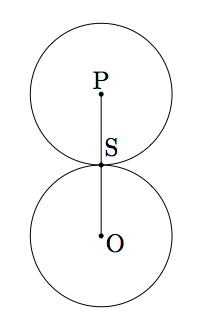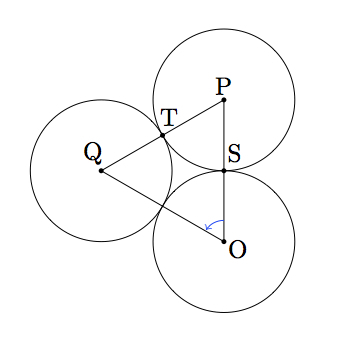# Seven Circles I

Alignments to Content Standards: G-SRT.C G-CO.C.10

Liz places seven pennies in the arrangement shown below:She notices that each of the six outer pennies touch the one in the center and its two outer neighbors. Liz wonders whether or not this is true if the pennies are replaced with seven nickels or quarters or, more generally, any set of seven equally sized circles.

What is the answer to Liz's question, that is, is it possible to place six circles around a central circle so that each outer circle touches the one in the center as well as its two outside neighbors?

## IM Commentary

This task is intended to help model a concrete situation with geometry. Placing the seven pennies in a circular pattern is a concrete and fun experiment which leads to a genuine mathematical question: does the physical model with pennies give insight into what happens with seven circles in the plane? The first solution uses the fact that the circles are the same size to show that the triangle made by connecting midpoints of three mutually adjacent circles is an equilateral triangle. The second solution studies the central circle and one circle in the outer ring, showing that six successive rotations through $60$ degrees, about the center of the central circle, bring the outer circle back to its original position.

As an open-ended task, this may be challenging but should provide a rich context for exploring properties of circles. The teacher may wish to provide some guidance toward finding a solution by suggesting to focus on the centers of the circles and the distances between centers of adjacent circles. An alternative solution uses rotations and relates to trigonometry: this second solution reveals some of the symmetry aspects of the seven circles which is further pursued in Seven Circles II. Another natural question which arises in working through this task is what happens if the circle on the inside is not the same size as those on the outside: for example, what if a dime or a quarter is placed in the middle? This question is examined in Seven Circles III.

The three dimensional analogue of this problem, where the circles are replaced by spheres, is even more interesting and has applications to chemistry as atoms are frequently modeled by spheres.

## Solutions

Solution: 1 Geometry

The seven circles all have the same size so we will let $r$ represent the common radius of all of the circles. If each outer circle touches the inner circle as appears to be the case in the picture, the distance between the center of an outer circle and the center of the inner circle must be $2r$. Similarly, the distance between centers of adjacent circles on the outside must be $2r$. This means that we would have six equilateral triangles, pictured below, formed by the center of the inner circle and the six centers of the outer circles:There are 360 degrees in a circle so the circle can be divided into $6$ angles each measuring $60$ degrees. Choose three circles with equal radii, as pictured below, where we assume that the measure of angle $POQ$ is $60$ degrees.Since the measure of angle $POQ$ is $60$ degrees and the triangle $POQ$ is isosceles by construction, the angles $OPQ$ and $OQP$ must also have a measure of $60$ degrees. Thus triangle $POQ$ is equilateral. It follows that $|PQ| = 2r$ and that these two circles meet in exactly one point, the midpoint of segment $PQ$. Similar reasoning shows that each pair of adjacent circles in the design touch in a single point so Liz's observation is correct.

Solution: 2 Rotations

Suppose we start with two circles, as pictured below, having the same radius $r$ and sharing a common point $S$.The point $S$ has distance $r$ from $P$ and distance $r$ from $O$. Suppose we rotate the circle with center $P$ by 60 degrees, counterclockwise, about $O$, producing the following picture:Since the rotation is through 60 degrees this means that $m(\angle POQ) = 60$. We know $\triangle POQ$ is isosceles with $|OP| = |OQ|$ because $\overline{OQ}$ is the image of $\overline{OP}$ by a rotation. This means that $m(\angle QPO) = m(\angle PQO) = 60$ and so $\triangle POQ$ is equilateral. Since $|OP| = 2r$ this means that $|PQ| = 2r$. The midpoint $T$ of $\overline{PQ}$ has distance $r$ from the circle with center $P$ and the circle with center $Q$: the circles cannot share a second point $U$ since then $\triangle PQU$ would have side lengths $r,r,$ and $2r$ which is not possible.

This procedure can now be repeated, rotating the circle with center $Q$ by 60 degrees about $O$ to produce a third circle in the outer ring, meeting the circle with center $Q$ in one point. The sixth circle in the outer ring is produced by a 300 degree counterclockwise rotation of the circle with center $P$. It will meet the fifth circle in a single point and similarly for the circle with center $P$ since a 300 degree counterclockwise rotation is the same as a 60 degree clockwise rotation.